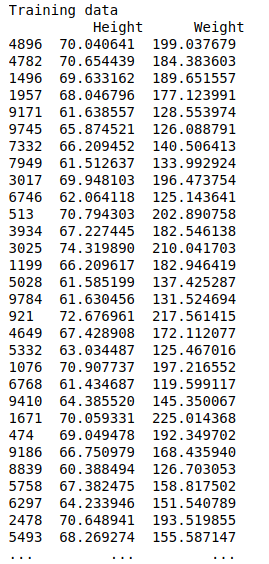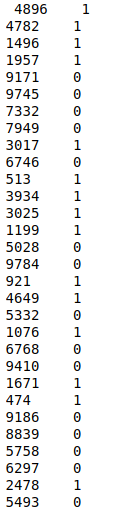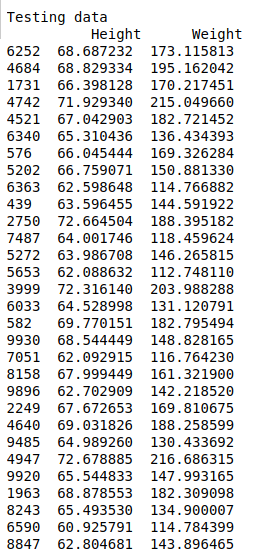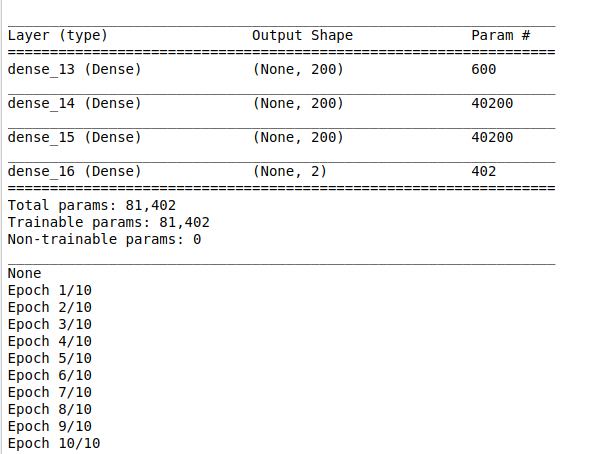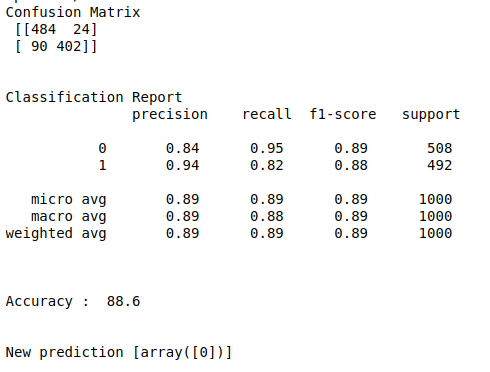• #5, First Floor, 4th Street Dr. Subbarayan Nagar Kodambakkam, Chennai-600 024 Landmark : Samiyar Madam
• pro@slogix.in
• +91- 81240 01111

### How to predict students gender using their height and weight data using Deep neural networks from keras in python?

###### Description

To build a DNN model to predict Gender of the students with help of keras in python.

###### Input

Height and Weight data set.

###### Output

Confusion matrix
Classification report
Accuracy score
Precision and recall

#### process:

Load the data set.

Define the independent and dependent variable.

Build the deep neural networks.

Initiate activation and optimizer functions according to the problem.

Compile and fit the DNN model.

Split the data into train and testing set.

Fit the training set into the model.

Predict the test results using DNN.

Calculate the accuracy, precision and recall.

###### Sample Code

#import necessary libraries
import time
import random
import warnings
warnings.filterwarnings(“ignore”)
import pandas as pd
import numpy as np
import matplotlib.pyplot as plt
import seaborn as sns
from keras.models import Sequential
from keras.layers import Dense
from sklearn.model_selection import train_test_split
from keras.utils import np_utils
from sklearn.metrics import classification_report, confusion_matrix

#load the data set

#Make it as a data frame
df = pd.DataFrame(data)

#Checking missing values
print(“Checking missing values\n”)
print(df.isnull().sum())

#Count plot for target variable
plt.rcParams[“figure.figsize”] = [9,6]
ax = sns.countplot(x=”Gender”,data=df)
plt.title(“Count plot for categorical variable”)
plt.show()
print(“\n”)

#Pre-processing
from sklearn import preprocessing

# label_encoder object knows how to understand word labels.
label_encoder = preprocessing.LabelEncoder()

# Encode labels in column ‘Gender’.
df[‘Gender’]= label_encoder.fit_transform(df[‘Gender’])

#feature selection
X = df.iloc[:,1:3]
y = df.iloc[:,0]

#Split the data into train and testing
X_train, X_test, Y_train, Y_test = train_test_split(X, y, test_size=0.1, random_state=42)

#Print training data
print(“Training data\n”,X_train,”\n”,Y_train)
print(“\n\n”)

#Print testing data
print(“Testing data\n”,X_test)
print(“\n\n”)

#make dependent variable categorical
Y_train = np_utils.to_categorical(Y_train,num_classes=2)
Y_test = np_utils.to_categorical(Y_test,num_classes=2)

#create model
model = Sequential()
shape = X_train.shape

#add layers to model
print(model.summary())

#Compile the model

#Here we train the Network.
model.fit(X_train, Y_train, batch_size=30, epochs = 10, verbose = 5)

#Here we evaluate the model
score,acc = model.evaluate(X_test,Y_test,verbose = 2,batch_size = 30)
#Predict the test results
prediction = model.predict(X_test)
length = len(prediction)
y_label = np.argmax(Y_test,axis=1)
predict_label = np.argmax(prediction,axis=1)

#classification report
print(“Confusion Matrix\n”,confusion_matrix(Y_test.argmax(axis=1),prediction.argmax(axis=1)))
print(“\n”)
print(“Classification Report\n”,classification_report(Y_test.argmax(axis=1),prediction.argmax(axis=1)))
print(“\n”)
accuracy = np.sum(y_label==predict_label)/length * 100
print(“Accuracy : “,accuracy)

#predict new value
print(“\n”)
new = [[68.01349487,120.45444004]]

#predict classes for test data
DNN_X_test = np.array(new)
DNN_y_pred = []
for i in range(0,len(new)):
DNN_y_pred.append(model.predict_classes(DNN_X_test[[i]]))
print(“New prediction”,DNN_y_pred)

###### Screenshots